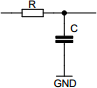# RC time constant calculator

## Calculates the time constant of a resistor-capacitor circuit. Time constant is the time required to charge or discharge the capacitor by ~63.2% of the difference between the initial and final value.

 Example 1: Must calculate the time constant of a 47uF capacitor and 22 ohm resistor View example
 Capacitance (C) farads Resistance (R) ohms Time constant secondsYou may use one of the following SI prefix after a value: p=pico, n=nano, u=micro, m=milli, k=kilo, M=mega, G=giga

Fill in all values except the one you wish to calculate
• Capacitance - Capacitance of the capacitor
• Resistance - Resistance of the resistor
• Time - Time constant of the circuit